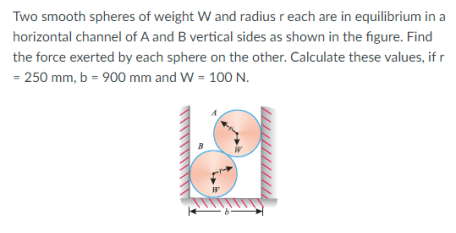# Two smooth spheres of weight W and radius r each are in equilibrium in a horizontal channel of A and B vertical sides as shown in the figure. Find the force exerted by each sphere on the other. Calculate these values, if r=250mm, b=900mm and W=100N.

Question-AnswerCategory: Engineering MechanicsTwo smooth spheres of weight W and radius r each are in equilibrium in a horizontal channel of A and B vertical sides as shown in the figure. Find the force exerted by each sphere on the other. Calculate these values, if r=250mm, b=900mm and W=100N.

### Two smooth spheres of weight W and radius r each are in equilibrium in a horizontal channel of A and B vertical sides as shown in the figure. Find the force exerted by each sphere on the other. Calculate these values, if r=250mm, b=900mm and W=100N.